The Sundial Primer created by Carl SabanskiThe Sundial Primer Index
 Gnomon Height Style: is the line in space which generates the shadow edge used to indicate the time on the dial plate.Nodus: is a point which casts a shadow to indicate the time and/or date on a dial plate.Declination Lines: are lines on a dial showing the sun's declination on a particular date.Figure 1: The parts of a sundial - Northern & Southern Hemispheres. The question is : How high should the gnomon be?  Or perhaps how long should the gnomon's style be?To answer this question you must  first make a few design decisions about your sundial. What are the dimensions of your dial plate? Will you have declination lines on the dial plate indicating the date? If declination lines exist, will they be traced by the upper tip of the style or will there be a nodus? If there will be no declination lines you must decide how short you want your shadow to get? It is at solar noon on the day of the summer solstice that the shadow cast by the gnomon of a horizontal sundial will be the shortest. At any other time and date the shadow will be longer. Where do you want this shadow to lie on your sundial or do you want it to extend beyond the north end of the dial plate? Perhaps you may have a gnomon already designed but you want to make sure that the shadow will always reach your hour line markers. You need to determine the length of the gnomon's shadow at solar noon on the summer solstice when the sun is at its maximum altitude.Figure 2: Summer Solstice Shadow Length Calculate the sun's maximum altitude: AMAX = (90 - Latitude +23.44)° Shadow Length = Gnomon Height / tan (AMAX) Table 1 lists the minimum shadow lengths (SMIN) at various latitudes based on a gnomon height of 1 unit.  When you determine the height of your gnomon, multiply by SMIN to determine the shortest shadow it will cast. If you notice the negative numbers at latitudes below 25° please go to the end of this page.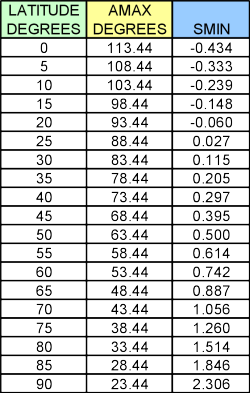Table 1: Minimum Shadow Lengths - Horizontal Sundial You can also determine the minimum shadow length that your gnomon will cast by using "The Dialist's Companion". This program allows you to set any location, date and time. The image below shows that the program has been set for June 20, 2004 and the "Dial Time" is set to 12:00 pm. To accomplish this, the "Pinawa Central" time was entered as 12:00 pm and then the "End" key was selected to freeze the screen. As long as the "End"  key is not selected again the "Pinawa Central" time can be adjusted to any time and the clock will remain frozen. Note that the time for "Solar Noon" is given as 12:25:12 pm. Entering this time will set the "Dial Time" to 12:00 pm. The length of the shadow is shown as "Shadow" and must be multiplied by the height of your gnomon.Figure 3: Determining minimum shadow length using "The Dialist's Companion". Perhaps you have no idea how large the gnomon should be. First establish the distance from the dial origin to the noon hour line marker. In Figure 4 this distance will be the sum of the gnomon "Base Length" and the "Shadow Length".Figure 4: How to Determine the Style Length - Horizontal Sundial By applying the Law of Sines, you can determine the style length as follows:Style Length / sin (Sun's Altitude) = (Base Length + Shadow Length) / sin 66.56 Style Length = 1.09 * (Base Length + Shadow Length) * sin (Sun's Altitude) Style Length = 1.09 * (Base Length + Shadow Length) * sin (113.44 - Latitude)Assuming a "Base Length + Shadow Length" of 1, Table 2 shows the required style length at various latitudes. Just multiply the "Style Length" factor by your required "Base Length + Shadow Length" and you will have the style length for your gnomon.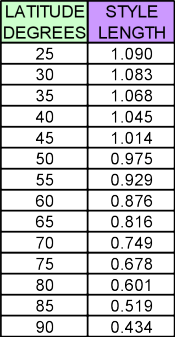Table 2: Calculating Style Length - Horizontal Sundial What about a vertical direct south sundial? It is at solar noon on the day of the winter solstice that the shadow cast by the gnomon of a vertical sundial will be the shortest. You need to determine the length of the gnomon's shadow at solar noon on the winter solstice when the sun is at its minimum altitude. Calculate the sun's minimum altitude: AMIN = (90 - Latitude - 23.44)° Shadow Length = Gnomon Height / tan (90-AMIN) Table 3 lists the minimum shadow lengths (SMIN) at various latitudes based on a gnomon height of 1 unit.  When you determine the height of your gnomon, multiply by SMIN to determine the shortest shadow it will cast.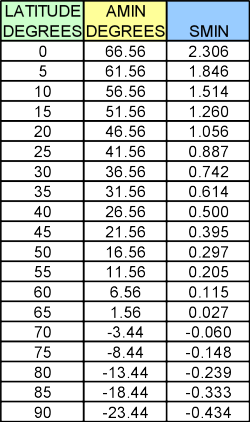Table 3: Minimum Shadow Lengths - Vertical Sundial To determine how large the gnomon should be first establish the distance from the dial origin to the noon hour line marker. In Figure 5 this distance will be the sum of the gnomon "Base Length" and the "Shadow Length".Figure 5: How to Determine the Style Length - Vertical Sundial By applying the Law of Sines, you can determine the style length as follows:Style Length / sin (90 - Sun's Altitude) = (Base Length + Shadow Length) / sin 66.56 Style Length = 1.09 * (Base Length + Shadow Length) * sin (90 - Sun's Altitude) Style Length = 1.09 * (Base Length + Shadow Length) * sin (Latitude + 23.44)Assuming a "Base Length + Shadow Length" of 1, Table 4 shows the required style length at various latitudes. Just multiply the "Style Length" factor by your required "Base Length + Shadow Length" and you will have the style length for your gnomon.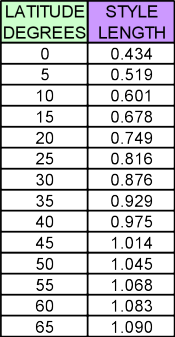Table 4: Calculating Style Length - Vertical Sundial Compare Table 1 to Table 3 and Table 2 to Table 4. Note that the values of "SMIN" in Table 1 and "STYLE LENGTH" in Table 2 for horizontal sundial at a particular latitude are the same as those in Tables 3 and 4 for a vertical direct south sundial at the co-latitude. What this indicates is that a vertical sundial at a particular latitude is the same as a horizontal sundial at the co-latitude. A vertical direct south sundial can be reduced to an equivalent horizontal sundial. The negative shadow length numbers in Table 1 occur in the tropics where the sun moves far enough north that shadows point toward the equator. Figure 6  is interesting and helps to visualize this occurrence. It is a graph of the "solar incidence angle" at local noon for various latitudes over the year. A solar incidence angle of 90° means that the sun is on the horizon and an angle of 0° means that it is directly overhead.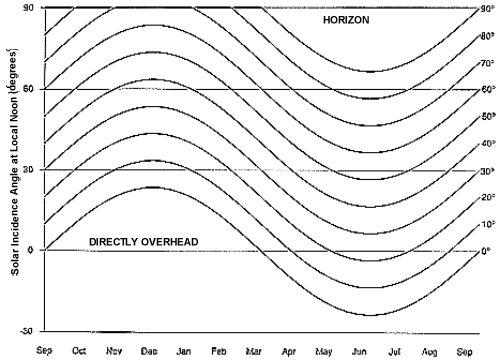Figure 6: Solar Incidence AngleNotice that at lower altitudes the sun is directly overhead twice a year. On those days a vertical rod will not cast a shadow. At higher latitudes the sun is never overhead and in the Arctic and Antarctic, the sun is on or below the horizon for part of the year. Check out the "Sun Charts" page for more interesting information.